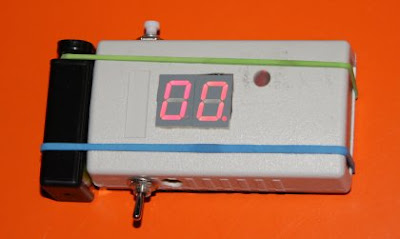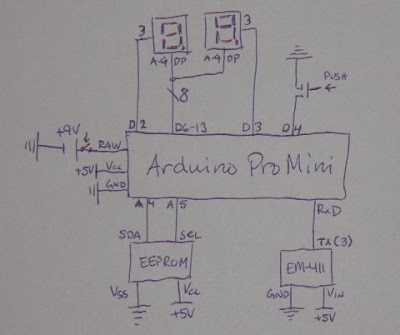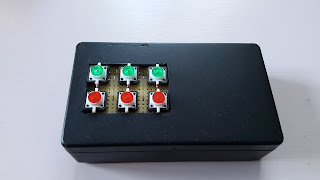## 2009-06-24

### A GPS logger with speedometer

To be able to contribute to the visionary OpenStreetMap project, I built myself a simple GPS logger. I added a speedometer as well, because I like to keep track of my speed when running or biking.I had a Globalsat EM-411 GPS module already, a leftover from one of my numerous unfinished projects. The EM-411 only does one thing, and that is to output NMEA0183 encoded messages using 4800bps RS-232 with TTL levels. No initialization or control messages necessary.

I used a 5V Arduino Pro Mini as microcontroller, because it is very easy to program with the simple IDE and Java-like language.

A 512Kbit EEPROM with I2C interface is used to store the GPS data. I could not get hold of a PDIP8 version, but I managed to solder a SO8 directly to a DIL socket for easier prototyping. There are a couple of useful references on how to control a I2C EEPROM from the Arduino. Make sure you connect it to ANALOG pins 4 & 5, which I did not do until after many hours of reading and debugging.

The speed is displayed using two seven segment displays. The decimal dots are used to show memory usage. Due to a minor thinking error, I used one with common anode and one with common kathode, but if you decide to use identical displays, this can easily be compensated for in the display() routine.

A push button trigger a memory dump to the serial port on the Arduino, which I connect to a PC using a TTL-232R cable from FTDI. The button can also be used to clear the memory, if pressed during power on.

With a three second log interval, the device can log for about an hour before the memory is full, and that is also the amount of time a 9V, 200mAh rechargeable battery will power it before going empty.

When a \$GPGGA message is received from the EM-411, it is stored in the EEPROM unaltered. The memory dump to PC is therefore just a plain playback of NMEA0183 messages. Using this approach, any NMEA0183 compatible software on the PC can read the logs without any conversions. I use GPSBabel to convert between NMEA0183 and GPX, the format required by OpenStreetMap.

When a \$GPRMC message is received from the EM-411, the speed in knots is extracted and converted to km/h before being displayed.`#include <Wire.h>#include <EEPROM.h>#define LOGDELAY 3000#define BUFLEN 100#define PHASEDELAY 10byte buttonPin = 4;byte commonPin = {3, 2};byte segmentPin = {9, 8, 7, 6, 13, 12, 11, 10};byte matrix = {1, 1, 1, 0, 1, 1, 1,                   1, 0, 0, 0, 0, 0, 1,                   1, 1, 0, 1, 1, 1, 0,                   1, 1, 0, 1, 0, 1, 1,                   1, 0, 1, 1, 0, 0, 1,                   0, 1, 1, 1, 0, 1, 1,                   0, 1, 1, 1, 1, 1, 1,                   1, 1, 0, 0, 0, 0, 1,                   1, 1, 1, 1, 1, 1, 1,                   1, 1, 1, 1, 0, 1, 1};int deviceaddress = 0x50;byte GGA = {'\$', 'G', 'P', 'G', 'G', 'A'};byte RMC = {'\$', 'G', 'P', 'R', 'M', 'C'};byte phase = 0;long nextphase = 0;int data = 0;int state = 0;unsigned int abase = 0;unsigned int logend = 0;byte packet[BUFLEN];byte length = 0;byte cache[BUFLEN];byte cachelength = 0;byte cachepos = 0;long time = 0;long nextlog = 0;void setup(){  Serial.begin(4800);  Wire.begin();    pinMode(commonPin, OUTPUT);  pinMode(commonPin, OUTPUT);  for (byte i = 0; i < 8; i++)  {    pinMode(segmentPin[i], OUTPUT);  }    pinMode(buttonPin, INPUT);  digitalWrite(buttonPin, HIGH); // Pull up    // Read memory pointers  abase = EEPROM.read(0);  abase <<= 8;  abase += EEPROM.read(1);  if (abase < 2 || abase > 510)  {    abase = 2;    EEPROM.write(0, abase >> 8);    EEPROM.write(1, abase & 0xFF);  }  logend = EEPROM.read(abase);  logend <<= 8;  logend += EEPROM.read(abase+1);  // Clear log if button pressed  if (digitalRead(buttonPin) == 0)  {    abase++;    if (abase > 510)    {      abase = 2;    }    EEPROM.write(0, abase >> 8);    EEPROM.write(1, abase & 0xFF);    logend = 0;    EEPROM.write(abase, logend >> 8);    EEPROM.write(abase+1, logend & 0xFF);          while (digitalRead(buttonPin) == 0);  }  }void loop(){  time = millis();    // Display driver  if (time > nextphase) {    display();    nextphase = time + PHASEDELAY;  }  // Dump log to serial port  if (digitalRead(buttonPin) == 0)  {    unsigned int address;    for (address = 0; address < logend; address++)    {      Serial.print(i2c_eeprom_read_byte(deviceaddress, address), BYTE);    }    if (digitalRead(buttonPin) == 0)    {      for (; address < 65535; address++)      {        Serial.print(i2c_eeprom_read_byte(deviceaddress, address), BYTE);      }      while (digitalRead(buttonPin) == 0);    }  }  // Serial receive  if (Serial.available() > 0)  {    int serialByte = Serial.read();    packet[length++] = serialByte;    if (serialByte == 10 || length >= BUFLEN)    {      parsePacket();      length = 0;    }  }    // EEPROM write  if (cachepos > 0)  {    i2c_eeprom_write_byte(deviceaddress, logend+cachepos, cache[cachepos]);    cachepos++;    if (cachepos == cachelength)    {      logend += cachelength;      EEPROM.write(abase, logend >> 8);      EEPROM.write(abase+1, logend & 0xFF);      cachepos = 0;      cachelength = 0;    }  } }void parsePacket(){  if (isGGA() && time > nextlog && cachepos == 0 && length > 50)  {    nextlog = time + LOGDELAY;        if (logend > 65535-length)    {      state = 3;      return;    }    else if (logend > 32768)    {      state = 2;    }    else    {      state = 1;    }    byte pos;    for (pos = 0; pos < length; pos++)    {      cache[pos] = packet[pos];    }    cachelength = length;    i2c_eeprom_write_byte(deviceaddress, logend+cachepos, cache[cachepos]);    cachepos++;  }  else if (isRMC())  {    byte pos = find(0, ',', 7);    float speed = parseFloat(pos);    data = speed * 1.852 + 0.5;  }}int parseInt(byte pos){  int result = 0;  while (packet[pos] >= '0' && packet[pos] <= '9')  {    result = result*10 + packet[pos] - '0';    pos++;  }  return result;}float parseFloat(byte pos){  float result = parseInt(pos);  pos = find(pos, '.', 1);  float decimals = parseInt(pos);  byte end = find(pos, ',', 1);  pos++;  while (pos < end)  {    decimals /= 10;    pos++;  }  return result + decimals;}byte find(byte pos, char sign, byte ncomma){  while (ncomma > 0)  {    if (packet[pos] == sign)    {      ncomma--;    }    pos++;  }  return pos;}boolean isGGA(){  for (byte i = 0; i < 6; i++)  {    if (GGA[i] != packet[i])    {      return false;    }  }  return true;}boolean isRMC(){  for (byte i = 0; i < 6; i++)  {    if (RMC[i] != packet[i])    {      return false;    }  }  return true;}void display(){  byte digit = 0;  digitalWrite(commonPin, 0);  digitalWrite(commonPin, 1);      if (phase == 0)  {    if (state & 0x02)    {      digitalWrite(segmentPin, 1);          }    else    {      digitalWrite(segmentPin, 0);                }    digit = (data % 100) / 10;  }  else  {    if (state & 0x01)    {      digitalWrite(segmentPin, 0);          }    else    {      digitalWrite(segmentPin, 1);                }    digit = data % 10;  }  for (byte i = 0; i < 7; i++)  {    digitalWrite(segmentPin[i], (phase ^ matrix[digit * 7 + i]) & 1);  }    if (phase == 0)  {    digitalWrite(commonPin, 0);    phase = 1;  }  else  {    digitalWrite(commonPin, 1);    phase = 0;  } }void i2c_eeprom_write_byte( int deviceaddress, unsigned int eeaddress, byte data ) {  Wire.beginTransmission(deviceaddress);  Wire.send((int)(eeaddress >> 8)); // MSB  Wire.send((int)(eeaddress & 0xFF)); // LSB  Wire.send((int)data);  Wire.endTransmission();  delay(10);}// WARNING: address is a page address, 6-bit end will wrap around// also, data can be maximum of about 30 bytes, because the Wire library has a buffer of 32 bytesvoid i2c_eeprom_write_page( int deviceaddress, unsigned int eeaddresspage, byte* data, byte length ) {  Wire.beginTransmission(deviceaddress);  Wire.send((int)(eeaddresspage >> 8)); // MSB  Wire.send((int)(eeaddresspage & 0xFF)); // LSB  byte c;  for ( c = 0; c < length; c++)    Wire.send(data[c]);  Wire.endTransmission();  delay(10);}byte i2c_eeprom_read_byte( int deviceaddress, unsigned int eeaddress ) {  byte rdata = 0x0F;  Wire.beginTransmission(deviceaddress);  Wire.send((int)(eeaddress >> 8)); // MSB  Wire.send((int)(eeaddress & 0xFF)); // LSB  Wire.endTransmission();  Wire.requestFrom(deviceaddress,1);  if (Wire.available()) rdata = Wire.receive();  return rdata;}// maybe let's not read more than 30 or 32 bytes at a time!void i2c_eeprom_read_buffer( int deviceaddress, unsigned int eeaddress, byte *buffer, int length ) {  Wire.beginTransmission(deviceaddress);  Wire.send((int)(eeaddress >> 8)); // MSB  Wire.send((int)(eeaddress & 0xFF)); // LSB  Wire.endTransmission();  Wire.requestFrom(deviceaddress,length);  int c = 0;  for ( c = 0; c < length; c++ )    if (Wire.available()) buffer[c] = Wire.receive();}`

From the code we can deduce that the two displays should be connected in parallel to digital I/O 6, 7, 8, 9, 10, 11, 12 and 13. Digital 2 and 3 are display select signals, connected to the anode and kathode on the displays. The push button is connected to digital 4, with the internal pull up resistor enabled. EM-411 TX (pin 3) is connected to Arduino RxD.